# Statistical Distributions

Every statistics book provides a listing of statistical distributions, with their properties, but browsing through these choices can be frustrating to anyone without a statistical background, for two reasons. First, the choices seem endless, with dozens of distributions competing for your attention, with little or no intuitive basis for differentiating between them. Second, the descriptions tend to be abstract and emphasize statistical properties such as the moments, characteristic functions and cumulative distributions. In this appendix, we will focus on the aspects of distributions that are most useful when analyzing raw data and trying to fit the right distribution to that data.

## Fitting the Distribution

When confronted with data that needs to be characterized by a distribution, it is best to start with the raw data and answer four basic questions about the data that can help in the characterization. The first relates to whether the data can take on only discrete values or whether the data is continuous; whether a new pharmaceutical drug gets FDA approval or not is a discrete value but the revenues from the drug represent a continuous variable. The second looks at the symmetry of the data and if there is asymmetry, which direction it lies in; in other words, are positive and negative outliers equally likely or is one more likely than the other. The third question is whether there are upper or lower limits on the data;; there are some data items like revenues that cannot be lower than zero whereas there are others like operating margins that cannot exceed a value (100%). The final and related question relates to the likelihood of observing extreme values in the distribution; in some data, the extreme values occur very infrequently whereas in others, they occur more often.

### Is the data discrete or continuous?

The first and most obvious categorization of data should be on whether the data is restricted to taking on only discrete values or if it is continuous. Consider the inputs into a typical project analysis at a firm. Most estimates that go into the analysis come from distributions that are continuous; market size, market share and profit margins, for instance, are all continuous variables. There are some important risk factors, though, that can take on only discrete forms, including regulatory actions and the threat of a terrorist attack; in the first case, the regulatory authority may dispense one of two or more decisions which are specified up front and in the latter, you are subjected to a terrorist attack or you are not.

With discrete data, the entire distribution can either be developed from scratch or the data can be fitted to a pre-specified discrete distribution. With the former, there are two steps to building the distribution. The first is identifying the possible outcomes and the second is to estimate probabilities to each outcome. As we noted in the text, we can draw on historical data or experience as well as specific knowledge about the investment being analyzed to arrive at the final distribution.  This process is relatively simple to accomplish when there are a few outcomes with a well-established basis for estimating probabilities but becomes more tedious as the number of outcomes increases. If it is difficult or impossible to build up a customized distribution, it may still be possible fit the data to one of the following discrete distributions:

a.     Binomial distribution: The binomial distribution measures the probabilities of the number of successes over a given number of trials with a specified probability of success in each try. In the simplest scenario of a coin toss (with a fair coin), where the probability of getting a head with each toss is 0.50 and there are a hundred trials, the binomial distribution will measure the likelihood of getting anywhere from no heads in a hundred tosses (very unlikely) to 50 heads (the most likely) to 100 heads (also very unlikely). The binomial distribution in this case will be symmetric, reflecting the even odds; as the probabilities shift from even odds, the distribution will get more skewed. Figure 6A.1 presents binomial distributions for three scenarios – two with 50% probability of success and one with a 70% probability of success and different trial sizes.

Figure 6A.1: Binomial Distribution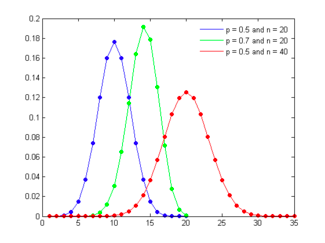As the probability of success is varied (from 50%) the distribution will also shift its shape, becoming positively skewed for probabilities less than 50% and negatively skewed for probabilities greater than 50%.

b.     Poisson distribution: The Poisson distribution measures the likelihood of a number of events occurring within a given time interval, where the key parameter that is required is the average number of events in the given interval (l). The resulting distribution looks similar to the binomial, with the skewness being positive but decreasing with l.  Figure 6A.2 presents three Poisson distributions, with l ranging from 1 to 10.

Figure 6A.2: Poisson Distribution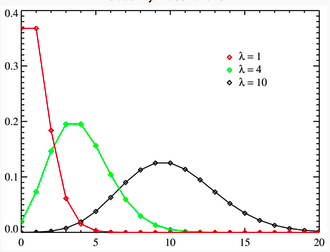c.     Negative Binomial distribution: Returning again to the coin toss example, assume that you hold the number of successes fixed at a given number and estimate the number of tries you will have before you reach the specified number of successes. The resulting distribution is called the negative binomial and it very closely resembles the Poisson. In fact, the negative binomial distribution converges on the Poisson distribution, but will be more skewed to the right (positive values) than the Poisson distribution with similar parameters.

d.     Geometric distribution: Consider again the coin toss example used to illustrate the binomial. Rather than focus on the number of successes in n trials, assume that you were measuring the likelihood of when the first success will occur. For instance, with a fair coin toss, there is a 50% chance that the first success will occur at the first try, a 25% chance that it will occur on the second try and a 12.5% chance that it will occur on the third try. The resulting distribution is positively skewed and looks as follows for three different probability scenarios (in figure 6A.3):

Figure 6A.3: Geometric Distribution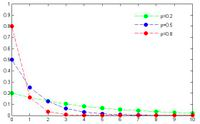Note that the distribution is steepest with high probabilities of success and flattens out as the probability decreases. However, the distribution is always positively skewed.

e.     Hypergeometric distribution: The hypergeometric distribution measures the probability of a specified number of successes in n trials, without replacement, from a finite population. Since the sampling is without replacement, the probabilities can change as a function of previous draws. Consider, for instance, the possibility of getting four face cards in hand of ten, over repeated draws from a pack. Since there are 16 face cards and the total pack contains 52 cards, the probability of getting four face cards in a hand of ten can be estimated. Figure 6A.4 provides a graph of the hypergeometric distribution:

Figure 6A.4: Hypergeometric Distribution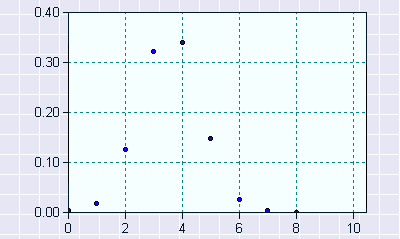Note that the hypergeometric distribution converges on binomial distribution as the as the population size increases.

f.      Discrete uniform distribution: This is the simplest of discrete distributions and applies when all of the outcomes have an equal probability of occurring.  Figure 6A.5 presents a uniform discrete distribution with five possible outcomes, each occurring 20% of the time:

Figure 6A.5: Discrete Uniform Distribution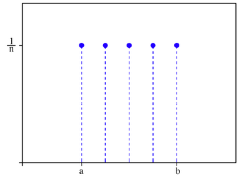The discrete uniform distribution is best reserved for circumstances where there are multiple possible outcomes, but no information that would allow us to expect that one outcome is more likely than the others.

With continuous data, we cannot specify all possible outcomes, since they are too numerous to list, but we have two choices. The first is to convert the continuous data into a discrete form and then go through the same process that we went through for discrete distributions of estimating probabilities. For instance, we could take a variable such as market share and break it down into discrete blocks – market share between 3% and 3.5%, between 3.5% and 4% and so on – and consider the likelihood that we will fall into each block. The second is to find a continuous distribution that best fits the data and to specify the parameters of the distribution. The rest of the appendix will focus on how to make these choices.

### How symmetric is the data?

There are some datasets that exhibit symmetry, i.e., the upside is mirrored by the downside. The symmetric distribution that most practitioners have familiarity with is the normal distribution, sown in Figure 6A.6, for a range of parameters:

Figure 6A.6: Normal Distribution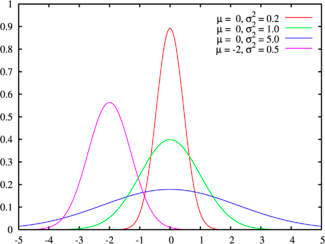The normal distribution has several features that make it popular. First, it can be fully characterized by just two parameters – the mean and the standard deviation – and thus reduces estimation pain. Second, the probability of any value occurring can be obtained simply by knowing how many standard deviations separate the value from the mean; the probability that a value will fall 2 standard deviations from the mean is roughly 95%.   The normal distribution is best suited for data that, at the minimum, meets the following conditions:

1. There is a strong tendency for the data to take on a central value.
2. Positive and negative deviations from this central value are equally likely
3. The frequency of the deviations falls off rapidly as we move further away from the central value.

The last two conditions show up when we compute the parameters of the normal distribution: the symmetry of deviations leads to zero skewness and the low probabilities of large deviations from the central value reveal themselves in no kurtosis.

There is a cost we pay, though, when we use a normal distribution to characterize data that is non-normal since the probability estimates that we obtain will be misleading and can do more harm than good. One obvious problem is when the data is asymmetric but another potential problem is when the probabilities of large deviations from the central value do not drop off as precipitously as required by the normal distribution. In statistical language, the actual distribution of the data has fatter tails than the normal. While all of symmetric distributions in the family are like the normal in terms of the upside mirroring the downside, they vary in terms of shape, with some distributions having fatter tails than the normal and the others more accentuated peaks.  These distributions are characterized as leptokurtic and you can consider two examples. One is the logistic distribution, which has longer tails and a higher kurtosis (1.2, as compared to 0 for the normal distribution) and the other are Cauchy distributions, which also exhibit symmetry and higher kurtosis and are characterized by a scale variable that determines how fat the tails are. Figure 6A.7 present a series of Cauchy distributions that exhibit the bias towards fatter tails or more outliers than the normal distribution.

Figure 6A.7: Cauchy DistributionEither the logistic or the Cauchy distributions can be used if the data is symmetric but with extreme values that occur more frequently than you would expect with a normal distribution.

As the probabilities of extreme values increases relative to the central value, the distribution will flatten out. At its limit, assuming that the data stays symmetric and we put limits on the extreme values on both sides, we end up with the uniform distribution, shown in figure 6A.8:

Figure 6A.8: Uniform Distribution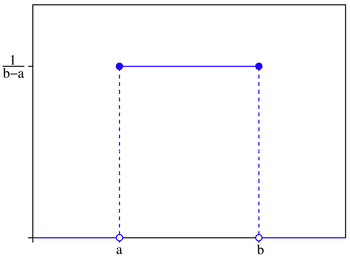When is it appropriate to assume a uniform distribution for a variable? One possible scenario is when you have a measure of the highest and lowest values that a data item can take but no real information about where within this range the value may fall. In other words, any value within that range is just as likely as any other value.

Most data does not exhibit symmetry and instead skews towards either very large positive or very large negative values. If the data is positively skewed, one common choice is the lognormal distribution, which is typically characterized by three parameters: a shape (s or sigma), a scale (m or median) and a shift parameter (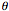). When m=0 and=1, you have the standard lognormal distribution and when=0, the distribution requires only scale and sigma parameters. As the sigma rises, the peak of the distribution shifts to the left and the skewness in the distribution increases. Figure 6A.9 graphs lognormal distributions for a range of parameters:

Figure 6A.9: Lognormal distribution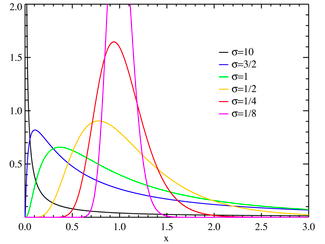The Gamma and Weibull distributions are two distributions that are closely related to the lognormal distribution; like the lognormal distribution, changing the parameter levels (shape, shift and scale) can cause the distributions to change shape and become more or less skewed. In all of these functions, increasing the shape parameter will push the distribution towards the left. In fact, at high values of sigma, the left tail disappears entirely and the outliers are all positive. In this form, these distributions all resemble the exponential, characterized by a location (m) and scale parameter (b), as is clear from figure 6A.10.

Figure 6A.10: Weibull DistributionThe question of which of these distributions will best fit the data will depend in large part on how severe the asymmetry in the data is. For moderate positive skewness, where there are both positive and negative outliers, but the former and larger and more common, the standard lognormal distribution will usually suffice. As the skewness becomes more severe, you may need to shift to a three-parameter lognormal distribution or a Weibull distribution, and modify the shape parameter till it fits the data. At the extreme, if there are no negative outliers and the only positive outliers in the data, you should consider the exponential function, shown in Figure 6a.11:

Figure 6A.11: Exponential Distribution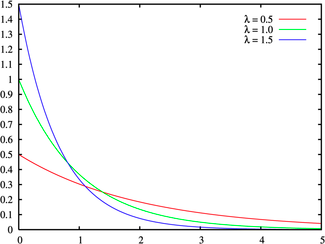If the data exhibits negative slewness, the choices of distributions are more limited. One possibility is the Beta distribution, which has two shape parameters (p and q) and upper and lower bounds on the data (a and b). Altering these parameters can yield distributions that exhibit either positive or negative skewness, as shown in figure 6A.12:

Figure 6A.12: Beta Distribution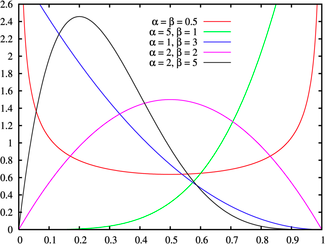Another is an extreme value distribution, which can also be altered to generate both positive and negative skewness, depending upon whether the extreme outcomes are the maximum (positive) or minimum (negative) values (see Figure 6A.13)

Figure 6A.13: Extreme Value Distributions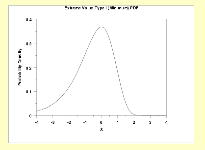### Are there upper or lower limits on data values?

There are often natural limits on the values that data can take on. As we noted earlier, the revenues and the market value of a firm cannot be negative and the profit margin cannot exceed 100%. Using a distribution that does not constrain the values to these limits can create problems. For instance, using a normal distribution to describe profit margins can sometimes result in profit margins that exceed 100%, since the distribution has no limits on either the downside or the upside.

When data is constrained, the questions that needs to be answered are whether the constraints apply on one side of the distribution or both, and if so, what the limits on values are. Once these questions have been answered, there are two choices. One is to find a continuous distribution that conforms to these constraints. For instance, the lognormal distribution can be used to model data, such as revenues and stock prices that are constrained to be never less than zero. For data that have both upper and lower limits, you could use the uniform distribution, if the probabilities of the outcomes are even across outcomes or a triangular distribution (if the data is clustered around a central value). Figure 6A.14 presents a triangular distribution:

Figure 6A.14: Triangular DistributionAn alternative approach is to use a continuous distribution that normally allows data to take on any value and to put upper and lower limits on the values that the data can assume. Note that the cost of putting these constrains is small in distributions like the normal where the probabilities of extreme values is very small, but increases as the distribution exhibits fatter tails.

### How likely are you to see extreme values of data, relative to the middle values?

As we noted in the earlier section, a key consideration in what distribution to use to describe the data is the likelihood of extreme values for the data, relative to the middle value. In the case of the normal distribution, this likelihood is small and it increases as you move to the logistic and Cauchy distributions. While it may often be more realistic to use the latter to describe real world data, the benefits of a better distribution fit have to be weighed off against the ease with which parameters can be estimated from the normal distribution. Consequently, it may make sense to stay with the normal distribution for symmetric data, unless the likelihood of extreme values increases above a threshold.

The same considerations apply for skewed distributions, though the concern will generally be more acute for the skewed side of the distribution. In other words, with positively skewed distribution, the question of which distribution to use will depend upon how much more likely large positive values are than large negative values, with the fit ranging from the lognormal to the exponential.

In summary, the question of which distribution best fits data cannot be answered without looking at whether the data is discrete or continuous, symmetric or asymmetric and where the outliers lie. Figure 6A.15 summarizes the choices in a chart.

## Tests for Fit

The simplest test for distributional fit is visual with a comparison of the histogram of the actual data to the fitted distribution. Consider figure 6A.16, where we report the distribution of current price earnings ratios for US stocks in early 2007, with a normal distribution superimposed on it.

Figure 6A.16: Current PE Ratios for US Stocks – January 2007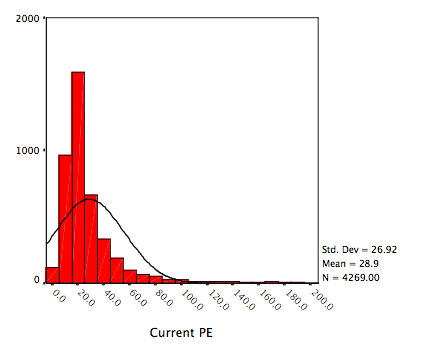The distributions are so clearly divergent that the normal distribution assumption does not hold up.

A slightly more sophisticated test is to compute the moments of the actual data distribution – the mean, the standard deviation, skewness and kurtosis – and to examine them for fit to the chosen distribution. With the price-earnings data above, for instance, the moments of the distribution and key statistics are summarized  in table 6A.1:

Table 6A.1: Current PE Ratio for US stocks – Key Statistics

 Current PE Normal Distribution Mean 28.947 Median 20.952 Median = Mean Standard deviation 26.924 Skewness 3.106 0 Kurtosis 11.936 0

Since the normal distribution has no skewness and zero kurtosis, we can easily reject the hypothesis that price earnings ratios are normally distributed.

The typical tests for goodness of fit compare the actual distribution function of the data with the cumulative distribution function of the distribution that is being used to characterize the data, to either accept the hypothesis that the chosen distribution fits the data or to reject it. Not surprisingly, given its constant use, there are more tests for normality than for any other distribution. The Kolmogorov-Smirnov test is one of the oldest tests of fit for distributions, dating back to 1967. Improved versions of the tests include the Shapiro-Wilk and Anderson-Darling tests. Applying these tests to the current PE ratio yields the unsurprising result that the hypothesis that current PE ratios are drawn from a normal distribution is roundly rejected:

Tests of Normality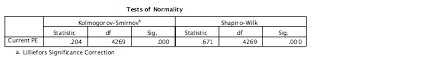There are graphical tests of normality, where probability plots can be used to assess the hypothesis that the data is drawn from a normal distribution. Figure 6A.17 illustrates this, using current PE ratios as the data set.Given that the normal distribution is one of easiest to work with, it is useful to begin by testing data for non-normality to see if you can get away with using the normal distribution. If not, you can extend your search to other and more complex distributions.

# Conclusion

Raw data is almost never as well behaved as we would like it to be. Consequently, fitting a statistical distribution to data is part art and part science, requiring compromises along the way. The key to good data analysis is maintaining a balance between getting a good distributional fit and preserving ease of estimation, keeping in mind that the ultimate objective is that the analysis should lead to better decision. In particular, you may decide to settle for a distribution that less completely fits the data over one that more completely fits it, simply because estimating the parameters may be easier to do with the former. This may explain the overwhelming dependence on the normal distribution in practice, notwithstanding the fact that most data do not meet the criteria needed for the distribution to fit.

#As the number of trials increases and the probability of success is close to 0.5, the binomial distribution converges on the normal distribution.

 The Kolgomorov-Smirnov test can be used to see if the data fits a normal, lognormal, Weibull, exponential or logistic distribution.